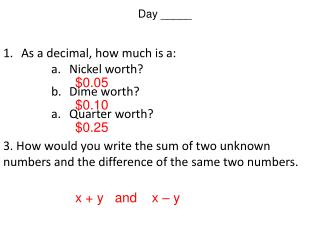DownloadDownload PresentationDay _____

# Day _____

Télécharger la présentation## Day _____

- - - - - - - - - - - - - - - - - - - - - - - - - - - E N D - - - - - - - - - - - - - - - - - - - - - - - - - - -
##### Presentation Transcript

1. Day _____ • As a decimal, how much is a: • Nickel worth? • Dime worth? • Quarter worth? • 3. How would you write the sum of two unknown numbers and the difference of the same two numbers. \$0.05 \$0.10 \$0.25 x + y and x – y

2. Coin & Number Word Problems I can write and solvesystems of linear equations word problems. Don’t forget to write this “I Can” in your target sheet.

3. Coin & Number Word Problems The sum of two numbers is 45, their difference is 7. Find the smaller of the two numbers. Ex 1. 1. Define your variables. Let x be one of the numbers and y be the other. 2. Create your equations. 3. Solve using elimination. + 4. Substitute to find other variable. 5. Answer the question. The smaller number is 19.

4. Ex 2. A jar of nickels and dimes contains \$8.60. There are 4 more nickels than dimes. How many of each coin is there? How much is a nickel worth? A dime? 1. Define your variables. Let x = # of nickels and y = # of dimes. Nickel is worth \$0.05 and a dime is worth \$0.10 2. Create your “money” equation. 3. Create your “# of each” equation. 4. Solve using any method. (substitution is probably best here) 5. Answer the question. There are 60 nickels and 56 dimes.

5. Ex 3. Julia smashed a piggy bank and found \$13.00 worth of nickels and quarters. There were 132 coins in all. How many quarters were there? How much is a nickel worth? A quarter? 1. Define your variables. Let x = # of nickels and y = # of quarters. Nickel is worth \$0.05 and a quarter is worth \$0.25 2. Create your “money” equation. 3. Create your “# of each” equation. 4. Solve using any method. + 5. Answer the question. There are 32 quarters in the piggy bank.

6. Ex 4. There were 500 tickets sold for a school play. Tickets for students were \$2 and adults were \$5. The total amount of money collected was \$1,900. How many tickets of each kind were sold? 1. Define your variables. Let x = # of student tickets and y = # of adult tickets. 2. Create your “money” equation. 3. Create your “total #” equation. 4. Solve using any method. 5. Answer the question. + They sold 200 student tickets and 300 adult tickets.